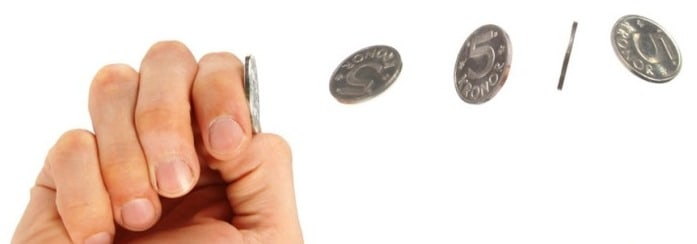Find Probability of 64th Head in 99th Toss of Fair CoinLet’s discuss how to find probability using multiplication rule and classical definition of probability. Consider the following problem. Suppose we’re asked to find a probability of obtaining $64^{th}$ head in the $99^{th}$ toss of fair coin. At the first glance the problem seems rather complicated, doesn’t it? Analyze carefully this question. In fact, we can divide the problem into two stages:

1) we managed to get $63$ heads in $98$ tosses;

2) we expect to obtain $64^{th}$ head in $99^{th}$ toss.

If both these situations occur then we get exactly the $64^{th}$ head in the $99^{th}$ toss. This means that the required probability is calculated as a product of the probabilities of two above situations. let’s name the probability of the first situations as $P_1$ and of the second – as $P_2$, then the probability we’re asked about is the following:

$P=P_1 \times P_2$

Check video-version of this tutorial on our channel:

So, all we need to do now is to find $P_1$ and $P_2$. The second probability can be found quite easily. Let’s have a closer look. In this situation ( we expect to obtain $64^{th}$ head in $99^{th}$ toss) we are tossing a coin once and we have only two possible outcomes, we get either head or tail. Obviously, the probability of having head in one toss of a fair coin is one half, therefore:

$P_2=0.5$

That’s it. Now move on and calculate the probability $P_1$ which is slightly more difficult. It goes as follows:

$P_1=\frac{C_{98}^{63}}{2^{98}}$

Again, we already have $63$ heads in $98$ tosses, that’s why we have $C_{98}^{63}$ in the numerator. The number $2^{98}$ in the denominator is the total amount of outcomes. Recall that due to classical definition, probability of the event is number of favorable outcomes over total number of outcomes. Thus, we have:

$P_1=\frac{C_{98}^{63}}{2^{98}}=\frac{\frac{98!}{63!(98-63)!}}{2^{98}}=\frac{98!}{63!35!2^{98}}$

Finally, let’s calculate the required probability:

$P=P_1 \times P_2=\frac{98!}{63!35!2^{98}} \cdot 0.5=\frac{98!}{63!35!2^{98}} \cdot \frac{1}{2}=\frac{98!}{63!35!2^{99}} \approx 0.00073$

As you can see, the probability of having the 64th head on the 99th toss of fair coin is pretty small. Have your own math questions? Don’t hesitate to ask, we help.

P.S. And now you can answer that for sure!5 Shares
Filed under Math.